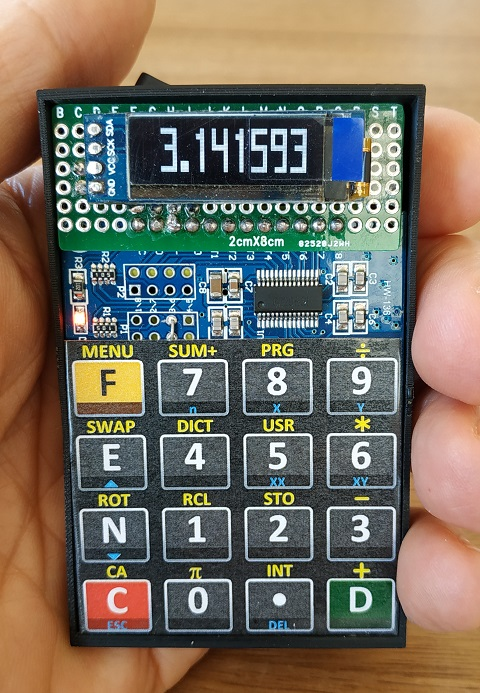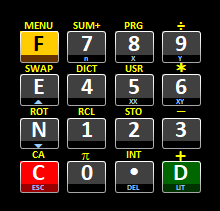{{ message }}

# zooxo / ivt Public

IVT (IV-TINY) - A FORTH-programable Scientific RPN Calculator that fits in 8 kilobytes (Arduino, ATTINY85)

Switch branches/tags
Nothing to show

## Files

Failed to load latest commit information.
Type
Name
Commit time
Jan 19, 2021
Mar 22, 2021
Mar 22, 2021

# IVT (IV-TINY) - A FORTH-programable Scientific RPN Calculator that fits in 8 kilobytes (Arduino, ATTINY85)See a short video of IVEE at: https://youtu.be/VqkXdZuKv6A

PREAMBLE

IVT (IVEE-TINY) is the smallest member of the IV-calculator series (FORTH-like programable calculators). The name Ivee or IV stands for the roman number 4, which was the basis for naming FORTH (4th generation programming language).

The hardware is simple:

• AVR ATTINY85 microcontroller (8192/512/512 bytes of FLASH/RAM/EEPROM)
• OLED-display (128x32, I2C, SSD1306)
• 16 keys touch sensitive keypad (TTP229-BSF, 2-wire)
• CR2032 battery

IVT was made without making compromises to offer a complete FORTH-like programming environment and a maximum of mathematical functions (mostly written in FORTH itself). And it's amazing, how much calculating power fits into 8 kilobytes:

• 80 intrinsic functions based on FORTH
• A wide range of mathematical and scientific commands (DUP DROP SWAP ROT OVER / * - + PI SQRT POWER INV INT EXP LN SIN COS TAN ASIN ACOS ATAN GAMMA P>R R>P nPr nCr)
• Statistics, line best fit and normal distibution (CDF/PDF)
• Present value calculations
• Programming: Up to 16 user definable programs with a total of 440 steps (< = <> > IF ELSE THEN BEGIN UNTIL)
• A solver to find roots of user defined functions
• A dictionary of all commands, words and programs
• Storing of 10 numbers/constants (permanently)
• Adjustable brightness of the display

Have fun! deetee

COMPILING

As IVT consumes 8190 bytes of flash memory (maximal 8192 bytes possible) compiling with proper settings is essential. Use the Arduino IDE, load the right library for the ATTINY85 and use the following settings:

• Library: "attiny by Davis A. Mellis"
• Chip: "ATtiny85"
• Clock: "8 MHz (internal)"
• B.O.D. Level: B.O.D. Disabled
• Save EEPROM: "EEPROM retained"
• Timer 1 Clock: "CPU (CPU frequency)"
• LTO: "Enabled"
• millis()/micros(): "Disabled"

KEYBOARD``````F(MENU) 7(SUM+) 8(PRG)  9(/)
E(SWAP) 4(DICT) 5(USR)  6(*)
N(ROT)  1(RCL)  2(STO)  3(-)
C(CA)   0(PI)   .(INT)  D(+)
``````

LIMITS

As a microprocessor is primarily not made to do such complex things like performing a powerful calculator there are some limits in performance and resources. Most obvious is the limited precision of the intrinsic float format (IEEE 754, 32 bit). As four bytes only are used to represent a float respective double number the decimal digits of precision are limited to 6...7.

In addition the resources of a microcontroller are limited like the FLASH memory (holds the executable program code), the RAM memory (holds variables and data while running) and the EEPROM (holds permanent data like settings or user programs).

Due to the target of maximal calculating power IVT lacks on many "features of comfort". For example there is no error control - a division by zero results in a "non interpretable" display.

However IVT tries to offer a maximum of features, comfort and performance with a minimum of required resources.

``````LIMITS:
24   ... Maximal data stack size
7    ... Maximum number of displayed significant digits of a number
10   ... Maximal amount of numbers saved permanently (0~9)
16   ... Maximal number of user programs
440  ... Maximal size (steps) of all user programs
32   ... Maximal definable command slots of user menu
``````

To navigate through the menu of some functions (MENU, DICT or USR) all selectable items are divided into four sections. Every section has its own up and down key (section I: E/N, section II: 4/1, section III: 5/2 and section IV: 6/3). To select one of the four presented items use the appropriate function key (F/7/8/9) or escape the menu with "C". There is some kind of hidden feature: If you leave the menu with the D-key the brightness of the display will be set (0~255, not permanent!).

COMMANDS

`````` BASIC KEYS:
0~9.     ... Digits and decimal point
EE N     ... 10-exponent (actually Y*10^X) and negate (change sign)
D        ... DUP (push stack) or complete number input
C        ... DROP top of stack or clear entry when in number input
F        ... Shift to select function keys or double press for user menu

FUNCTION KEYS:
+ - * /  ... Basic operations
SUM+     ... Enter X-data for statistics or X,Y-data for linear regression
PRG      ... Edit program (00~15) - enter program number first
SWAP     ... Swap the two top numbers of stack (1 2 -> 2 1)
DICT     ... Browse (and select) the complete dictionary
USR      ... Set dictionary entry to user menu
ROT      ... Rotate stack (1 2 3 -> 2 3 1)
STO RCL  ... Store/recall number - enter memory number first (0~9)
CA       ... Clear stack and memories for statistics (5~7)
PI       ... Push PI to stack
INT      ... Integer value

DICTIONARY (4 sections):
F  7  8  9   ... F-key, numbers
EE 4  5  6   ... 10-exponent, numbers
N  1  2  3   ... NEGATE, numbers
C  0  .  D   ... Clear/DROP, number, dot, DUP
M  S+ PR /   ... MENU, SUM+, PRG edit, divide

>< DC US *   ... SWAP, DICT, set USER menu, multiply
RT RC ST -   ... ROT, RCL, STO, subtract
CA PI IN +   ... CA (clear all), PI, INT, add
IF EL TH PC  ... IF, ELSE, THEN, nPr/nCr
<  =  <> >   ... LESSTHAN, EQUAL, NOTEQUAL, GREATERTHAN

BE UN SO I   ... BEGIN, UNTIL, SOLVE, INV (1/X)
c  t- E  LN  ... COS, ATAN, EXP, LN
s  t  s- c-  ... SIN, TAN, ASIN, ACOS
OV SQ yx !L  ... OVER, SQRT, POW (y^x), ln(GAMMA)
PV ND P> R>  ... Present value, normal distribution, P->R, R->P

S+ Sc x- LR  ... SUM+, SUMclr (clears memories 5~9), MEAN/STDEV, L.R.
00 01 02 03  ... User programs
04 05 06 07  ... User programs
08 09 10 11  ... User programs
12 13 14 15  ... User programs
``````

PV, ND, P<>R, STAT

``````PV     ... Present value of given interest rate (ie. 0.08) and periods
ND     ... PDF (X) and CDF (Y) of standard normal distribution
P> R>  ... Polar/Rectangular conversion
STAT   ... Mean value (X) and standard deviation (Y).
Note that the memories 5~9 (see RCL/STO) are used as statistic
registers (Sxx, Sxy, n, Sx, Sy).
LR     ... Line best fit (y = X * x + Y)
``````

PROGRAMMING

IVT is able to deal with up to 16 user programs (named 00-15) with a total number of 440 steps/commands. The maximal size per user program rises from 20 steps (program 00) to 35 steps (program 15). To edit a program, enter the program number (00-15) followed by PRG (F-8). The display shows P (for program), the program number (00-15), the program step number (vary with cursor keys E and N) and the command of this step. To insert a program step

• press a key (number or DUP),
• press a shifted key (press F twice to toggle) or
• press DICT (F-4) to select a command from the dictionary. To delete a program step press ".". Leave and save the program with "C". To execute a program select the appropriate program number/name from DICT. Please note that the first user program (00) will be used by the solver.

SOLVER

To find a root of a function (programmed in user program 00) enter an appropriate start value and select the command SO from the dictionary.

POWER CONSUMPTION

As IVT provides a maximum of calculating power there where less resources for a good power management (i.e. idle, screen saver, auto power off) left. Merely the not needed timer1 and AD-convertes are set off to save approximately 0.9 mA. The power consumption of IVT depends mainly on the usage of the display. That's why per default the brightness of the display is set to minimum. Please note that you can (not permanently) set the brightness of the display with pressing D when in any menu (takes brightness value from stack). In total IVT consumes approximately 9 mA - so a a single battery (CR2032) which has a capacity of approximately 200 mAh should theoretically work at least 20 hours. Electrical current drawn by device (approximately):

• ATTINY85 ... 5 mA
• Display ... 1~4 mA (promt ~ display full of 8's)

PROGRAM EXAMPLES

``````  ABS:   DUP 0 LT IF NEG THEN
FRAC:  DUP INT -
SINH:  EXP DUP INV NEG + 2 /          ... sinh=(exp(x)-exp(-x))/2
COSH:  EXP DUP INV + 2 /              ... cosh=(exp(x)+exp(-x))/2
TANH:  2 * EXP DUP 1 - SWAP 1 + /     ... tanh=(exp(2*x)-1)/(exp(2*x)+1)
ASINH: DUP DUP * 1 + SQRT + LN        ... asinh(x)=ln(x+sqrt(x*x+1))
ACOSH: DUP DUP * 1 - SQRT + LN        ... acosh(x)=ln(x+sqrt(x*x-1))
ATANH: DUP 1 + SWAP NEG 1 + / SQRT LN ... atanh(x)=ln(sqrt((1+x)/(1-x)))
POW10: 1 SWAP EE
LOG:   LN 1 0 LN /                    ... log(x)=ln(x)/ln(10)
%: OVER / 1 0 0 *                     ... %=x/B*100%
CHG%: OVER - OVER / 1 0 0 *           ... chg%=(x-B)/B*100%

QE: OVER 2 / DUP * SWAP - SQRT SWAP 2 / NEG
SWAP OVER OVER - ROT ROT +            ... x12=-p/2+-sqrt(p*p/4-q)

DEG<>RAD: DUP PI * 1 8 0 / SWAP 1 8 0 - * PI /
C<>F: DUP 1 . 8 * 3 2 + SWAP 3 2 - 1 . 8 /
KM<>MI: DUP 1 . 6 0 9 3 4 4 DUP DUP ROT SWAP / ROT ROT *
M<>FT: DUP 3 . 3 7 0 0 7 9 DUP DUP ROT * ROT ROT /
CM<>IN: DUP 2 . 5 4 DUP DUP ROT SWAP / ROT ROT *
KG<>LBS: DUP 2 . 2 0 4 6 2 3 DUP DUP ROT * ROT ROT /
L<>GAL:  DUP 3. 7 8 5 4 1 2 DUP DUP ROT SWAP / ROT ROT *

HMS2H: DOT 0 0 0 0 0 1 ADD // Round up to prevent leaps
DUP DUP INT SWAP OVER - 1 0 0 * INT // hh mm
ROT 3 PICK SUB 1 0 0 MULT OVER SUB 1 0 0 MULT, // ss
3 6 0 0 / SWAP 6 0 / + + // ->s ->h

H2HMS: DUP 3 6 0 0 * DUP ROT INT // h->s
SWAP OVER 3 6 0 0 * - 60 DIV INT, // hh mm
ROT OVER 6 0 MULT SUB 3 PICK 3 6 0 0 * - // ss
1 0 0 0 0 / SWAP 1 0 0 / + + // hh.mmss

nPr = n!/(n-r)! : OVER ROT ROT - 1 ROT ROT SWAP
BEGIN SWAP ROT 1 ROT + DUP ROT * SWAP ROT OVER OVER SWAP LT UNTIL DROP DROP

nCr = n!/(n-r)!/r! = nPr/r! : DUP ROT SWAP PERM 1 ROT
BEGIN ROT ROT DUP ROT SWAP / ROT ROT 1 + SWAP OVER 1 - OVER SWAP LT UNTIL DROP DROP
``````

ATTINY85 PINS

``````                      _____
Analog0/Reset P5 H1|* U  |H8     Vcc
Analog2 P3 H2|     |H7 P2  SCK/Analog1
Analog3 P4 H3|     |H6 P1  PWM1/MISO
GND    H4|_____|H5 P0  PWM0/AREF/SDA/MOSI
``````

CIRCUIT DIAGRAM

`````` _________________
|   OLED-DISPLAY  |
|  128x32 SSD1306 |
|_GND_VCC_SCK_SDA_|
|   |   |   |
|   |
__|___|___|___|__
| GND VCC P2  P0  |
|  AVR  ATTINY85  |
|_________P3__P1__|
|   |
|   |
__|___|___|___|__
| VCC GND SCL SDO |
|-----------------|
|  O O O O        |
|                 |
|  O O O O        |
|  O O O O        |
|      |          |... Solder to enable
|  O O O O        |    16-key-mode (TP2=0)
|                 |
``````

IVT (IV-TINY) - A FORTH-programable Scientific RPN Calculator that fits in 8 kilobytes (Arduino, ATTINY85)